Value-Based Questions: Surface Areas & Volumes

# Value-Based Questions: Surface Areas & Volumes - Mathematics (Maths) Class 9

Question 1. Shanti Sweets stall placed an order to Mr. Kishorilal for making cardboard boxes for packing their sweets. Two sizes of boxes are ordered. The bigger of dimensions 25cm × 20cm × 5cm and the smaller of dimensions 15cm × 12cm × 5cm. For all the overlaps, 5% of the total surface is required extra. 250 boxes of each kind are required. Cost of the cardboard is ₹ 4 for 1000 cm2. By mistake Shanti Sweets Stall made payment according to the bigger boxes, but Mr. Kishorilal returned back the excess money.
(a) What amount was returned back by Mr. Kishorilal to the Shanti Sweets Stall?
(b) Which mathematical concept is used in the above problem?
(c) By returning back the access money, which value is depicted by Mr. Kishorilal?
Sol.
(a) Total surface area of 250 bigger boxes:variety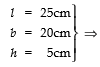Surface area of 1 box = 2 (lb + bh + hl)

= 2 (25 × 20 + 20 × 5 + 5 × 25) cm2
= 2 (500 + 100 + 125) cm
= 2 × 725 cm= 1450 cm
∴ Surface area of 250 boxes = 250 × 1450 cm
= 362500 cm2

Total surface area of 250 smaller boxes :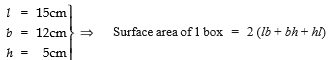= 2 (15×12 + 12×5 + 5×15) cm2
= 2 (180 + 60 + 75) cm2 = 630 cm2
∴ Total surface area of 250 boxes = 250 × 630 cm2 = 157500 cm2
Total surface area of both kinds of boxes = 362500 cm+ 157500 cm2 = 520000 cm2
Area for overlaping = 5% of 520000 cm2 = 26000 cm2
∴ Total surface area for which actual payment is to be made = 520000 cm2 + 26000 cm2 = 546000 cm2
Actual cost = (4/1000)× 546000 = ₹ 2184
But by mistake the payment is made for 500 boxes, each of dimension 25cm × 20cm × 5cm
∴ Total surface area 250 + 250 boxes for wrong payment = 500 × 2 [lb × bh × hl]
= 500 × 2 [25 × 20 + 20 × 5 + 5 × 25] cm2
= 500 × 1450 cm2 = 725000 cm2
Area for overlaping = 5% of 725000 = 36250 cm
Total surface area for wrong payment = (72500 + 36250) cm2 = 761250 cm
∴ Wrong payment made by the Shanti Sweets Stall =₹ (4/1000)× 761250 = ₹ 3045
⇒ Excess amount paid by Shanti Sweets Stall to Mr. Kishorilal = ₹ 3045 – ₹ 2184 = ₹ 861
(b) Surface Areas and Volumes.
(c) Honesty.

Question 2. Rahul plans to erect an indoor greenhouse (herbarium) which is made entirely of glass panes (including base) held together with tape. It is 30 cm long, 25 cm wide and 25 cm high.
(a) What is the surface area of the glass used?
(b) How much of tape is needed for all the 12 edges?
(c) Which mathematical concept is used in the above problem?
(d) By erecting a greenhouse, which skill is depicted by Rahul?
Sol
. The herbarium is in the form of a cuboid, having l = 30cm, b = 25cm and h = 25cm.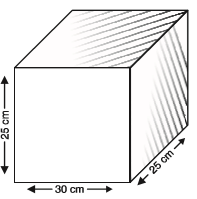(a) ∵ Surface area of the herbarium =2 [lb + bh + hl]
= 2 [(30 × 25) + (25 × 25) + (25 × 30)] cm2
= 2 [750 + 625 + 750] cm2
= 2  cm2 = 4250 cm2
⇒ The surface area of the herbarium = 4250 cm2

(b) Total length of 12 edges = 4l + 4b + 4h = 4 [l + b + h]
= 4 [30 + 25 + 25] cm
= 320 cm ⇒ 320 cm of tape is needed.

(c) Surface Areas and Volumes [Mensuration]

(d) Betterment of the environment.

Question 3. The Gram Panchayat of a village, plan to construct an underground cuboidal reservoir for water harvesting which is 2 km long, 40 m wide and 3 m deep. (a) Find the volume of water which can be stored in the reservoir.
(b) Which mathematical concept is used in the above problem?
(c) Which skill is depicted by the Gram Panchayat by constructing a water reservoir?
Sol.
(a) ∴ Dimensions of the cuboidal reservoir are: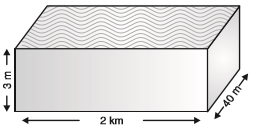l = 2 km = 2000 m b = 40 m and h = 3 m
∴ Volume (capacity) of the reservoir = l × b × h = 2000 × 40 × 3 m3 = 240000 m

(b) Mensuration [Surface Areas and Volumes]

(c) Protection of the natural resources.

Question 4. Rahul is having his birthday on coming Sunday. His father is planning to spend  3850.00 on his birthday. But Rahul has a different idea.
He wish to serve soup to patients in a hospital. The cost of 1 litre of soup is
50, it is served in a cylindrical bowl of diameter 7 cm. If the bowl is filled with soup to a height of 4 cm, then :
(a) How many patients can be served with soup?
(b) Which mathematical concept is used in the above problem?
(c) By spending is birthday money for patients, which values are depicted by Rahul?
Sol. (a)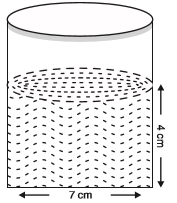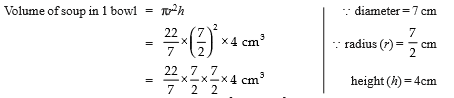= 11 × 7 × 2 cm3 = 154 cm
Let number of patients served = x
∴ Volume of soup served to x patients = 154 x cm3

Cost of 154 x cm3 soup =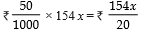⇒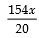= 3850

⇒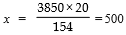⇒ Number of patients served with soup = 500

(b) Surface Areas and Volumes [mensuration]

(c)(i) Serving the patients.

(ii) Proper use of money.

Question 5. Nitin used to save a part of his pocket money. He wish to buy paint for a community-centre from his savings. He buys paint in a certain container which is sufficient to paint an area equal to 9.375 m2.
(a) How many bricks of dimensions 22.5 cm × 10 cm × 7.5 cm can be painted out of this container?
(b) Which mathematical concept is used in the above problem?
(c) By using the pocket-money saving to buy paints for community-centre, which values are depicted by Nitin?
Sol.
(a) Since, a brick is like a cuboid having l = 22.5 cm, b = 10 cm, h = 7.5 cm
∴ Total surface area of a brick = 2 [lb + bh + hl]
= 2 [(22.5 × 10) + (10 × 7.5) + (7.5 × 22.5)] cm
= 2 [225 + 75 + 168.75] cm2
= 2 [468.75] cm2 = 937.5 cm2
Let the required number of bricks = n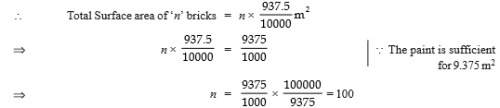Thus the required number of bricks = 100

(b) Surface Areas and Volumes [mensuration].

(c)(i) Saving money (ii) Charity.

Question 6. A welfare society plan to dig a rectangular tank for garbage-dumping purpose measuring 5m × 4.5m × 2.1m . The mud taken out of the above tank is purchased by Ankur, who spreads it evenly over his plot measuring 15m × 9m.
Ankur paid for the mud at the rate of
400 per cubic metre.
(a) Find the amount paid by Ankur to the welfare society.
(b) Which mathematical concept is used in the above problem?
(c) By digging a tank for ‘garbage-dumping’, what skill is depicted by the Welfare Society?
Sol.
(a)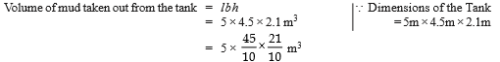The mud so taken out is spread over a base 15m × 9m.
Let height of the spreadover mud be ‘h’ metre.
∴ Volume of the mud = 15m × 9m × ‘h’ m3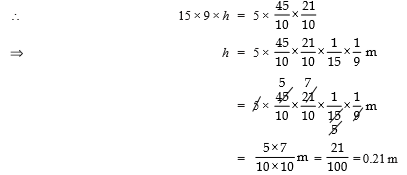Volume of mud = 15 m × 9 m × 0.21 m
Cost of mud = ₹ 400 × 15 × 9 × 0.21 = ₹ 11340
Thus, Ankur paid ₹ 11340 to welfare society.
(b) Areas and Volumes               [Mensuration]
(c) Betterment of the Environment.

Question 7. 35 students of class IX of a Vidyalya were asked to participate in a competition for making and decorating penholders in the shape of a cylinder with a base, using cardboard. Each penholder was to be of radius 3 cm and height 10.5 cm. The Vidyalya was to supply the competitors with cardboard.
(a) How much cardboard was required to be bought for the competition?
(b) Which mathematical concept is used in the above problem?
(c) By making cylindrical penholders and decorating them, which skills are depicted by the students of class IX?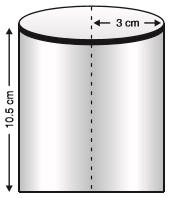Sol. (a) Here, the penholders are in the form of cylinders.
Radius of a cylinder (r) = 3 cm
Height of a cylinder (h) = 10.5 cm
Since, each penholder is open from the top
∴ Surface area of a  penholder (cylinder) = [Lateral surface area] + [Base area]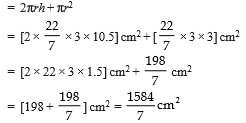⇒ Total surface area of 35 penholders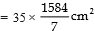= 5 × 1584 cm2 = 7920 cm2
Thus, 7920 cm2 of cardboard was required to be bought for 35 competitors.

(b) Surface Areas and Volumes

(c) Healthy competition and creativity.

Question 8. Students of class IX of the Sagar School plan to have their school bus stand barricaded from the remaining part of the road to avoid inconvenience of the people. For this purpose they use 50 hollow cones made of recycled cardboard. Each cone has a diameter of 40 cm and height 1m.
They painted outer side of each of the cones. The cost of painting is
12 per m2.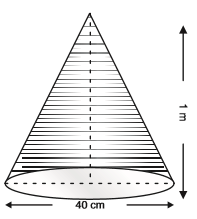[use π = 3.14 and √(1.04) = 1.02]

(a) Find the cost of painting of all these cones.

(b) Which mathematical concept is used in the above problem?

(c) By barricading the school-bus stand using cones of recycled cardboard, which skills are depicted by the students of class IX of the Sagar School?

Sol. (a) Diameter of a cone = 40 cm
∴ Radius of a cone (r)= 40
2 cm = 20 cm = 20 m 100
= 0.2 m Height of a cone (h)= 1m
⇒ Slant height of a cone (l)=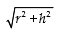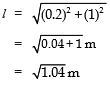= 1.02 m [∵ It is given that √(1.04)= 1.02]
Now, curved surface area of a cone = πrl                             [∵ Base of the cone is hollow]
⇒ Curved surface area of 1 cone = 3.14 × 0.2 × 1.02 m2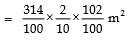⇒ Curved surface area of 50 cones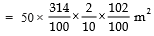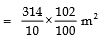Cost of Painting :
∵ The rate of painting is ₹ 12 per m2

∴ Total cost of 50 cones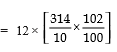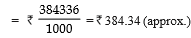(b) Surface Areas and Volumes [mensuration]
(c)(i) Care for the public convenience.
(ii) Betterment of environment.

Question 9. Rohit has set up a juice-shop. He has two types of packs : Type A : A cylindrical tin cane with circular base of diameter 7 cm and height 10 cm.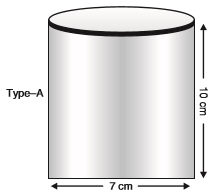Type B: A rectangular plastic cane with base-length 5 cm and width 4 cm and having height as 15 cm.
He decided to serve the customers in ‘A’ type of packs.

(a) Find the volume of pack ‘A’.

(b) Which pack has the greater capacity?

(c) Which mathematical concept is used in above problem?

(d) By choosing a pack of type-A and avoiding a plastic pack, which values are depicted by Rohit?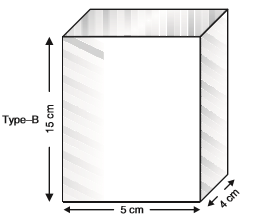Sol. (a) Volume of the cylindrical pack :
∵ Base diameter = 7 cm
2 cm Height (h) = 10 cm
∵ Volume = πr2h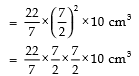⇒ = 11 × 7 × 5 cm3 = 385 cm
⇒ Volume of the cylindrical pack is 385 cm3

(b) Volume of rectangular pack : Length

(b) = 4 cm Height (h) = 15 cm
∴ Volume = lbh = 5 × 4 × 15 cm3 = 300 cm3
Since, 385 cm3 > 300 cm
⇒ Capacity of cylindrical pack is greater.
(c) Surface Areas and Volumes [mensuration]
(d)(i) Honesty (ii) Betterment of environment.

The document Value-Based Questions: Surface Areas & Volumes | Mathematics (Maths) Class 9 is a part of the Class 9 Course Mathematics (Maths) Class 9.
All you need of Class 9 at this link: Class 9

## FAQs on Value-Based Questions: Surface Areas & Volumes - Mathematics (Maths) Class 9

 1. What is the formula for finding the surface area of a rectangular prism?Ans. The formula for finding the surface area of a rectangular prism is given by 2(lw + lh + wh), where l, w, and h represent the length, width, and height of the prism, respectively.
 2. How can we find the surface area of a cylinder?Ans. The surface area of a cylinder can be found by adding the areas of its curved surface and two circular bases. The formula for the surface area of a cylinder is 2πrh + 2πr^2, where r represents the radius of the base and h represents the height of the cylinder.
 3. What is the formula for finding the volume of a cone?Ans. The formula for finding the volume of a cone is given by (1/3)πr^2h, where r represents the radius of the base and h represents the height of the cone.
 4. How do we calculate the surface area of a sphere?Ans. The surface area of a sphere can be calculated using the formula 4πr^2, where r represents the radius of the sphere.
 5. What is the formula for finding the volume of a rectangular prism?Ans. The formula for finding the volume of a rectangular prism is given by V = lwh, where l, w, and h represent the length, width, and height of the prism, respectively.

## Mathematics (Maths) Class 9

62 videos|426 docs|102 tests

## Mathematics (Maths) Class 9

62 videos|426 docs|102 tests
Signup to see your scores go up within 7 days! Learn & Practice with 1000+ FREE Notes, Videos & Tests.
10M+ students study on EduRev
Track your progress, build streaks, highlight & save important lessons and more!(Scan QR code)
Related Searches

,

,

,

,

,

,

,

,

,

,

,

,

,

,

,

,

,

,

,

,

,

;